•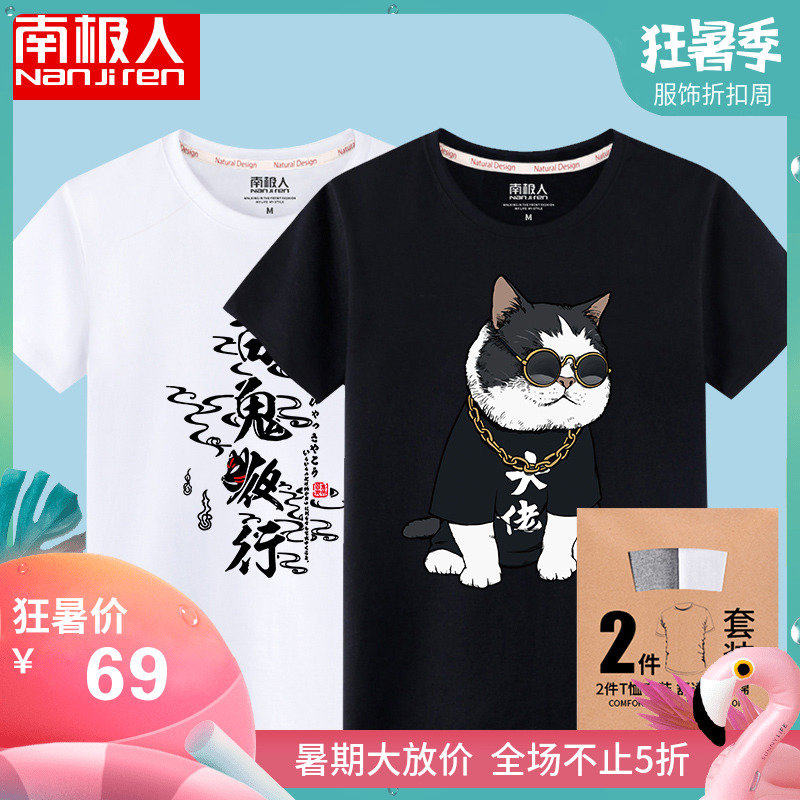••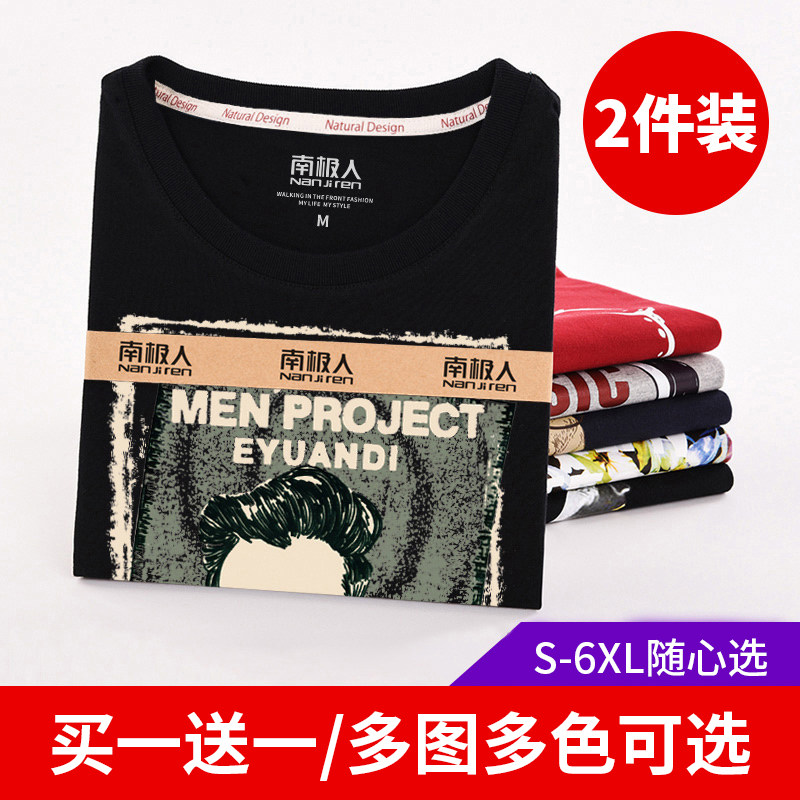•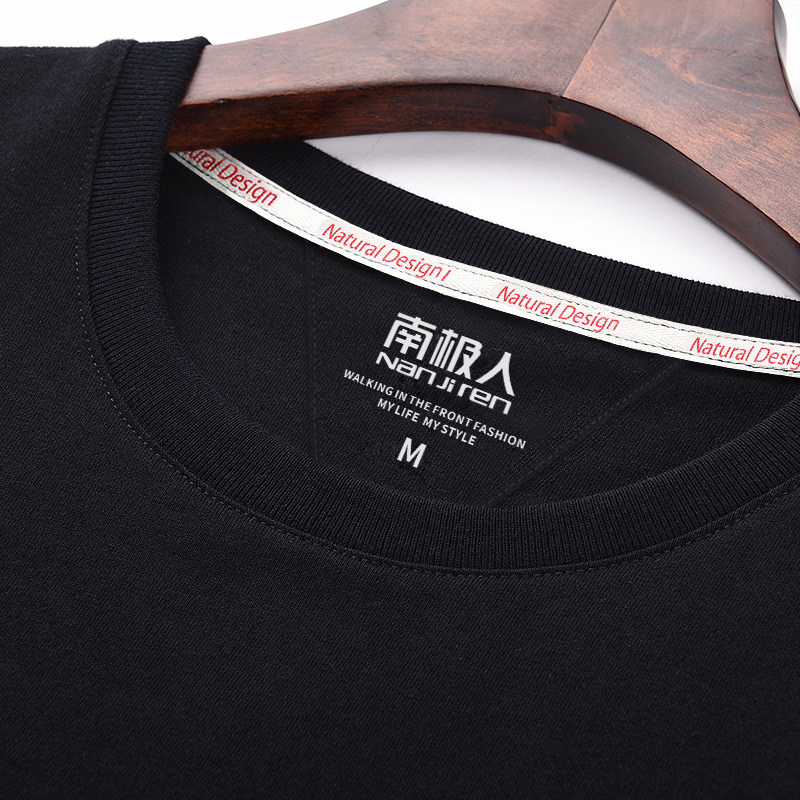•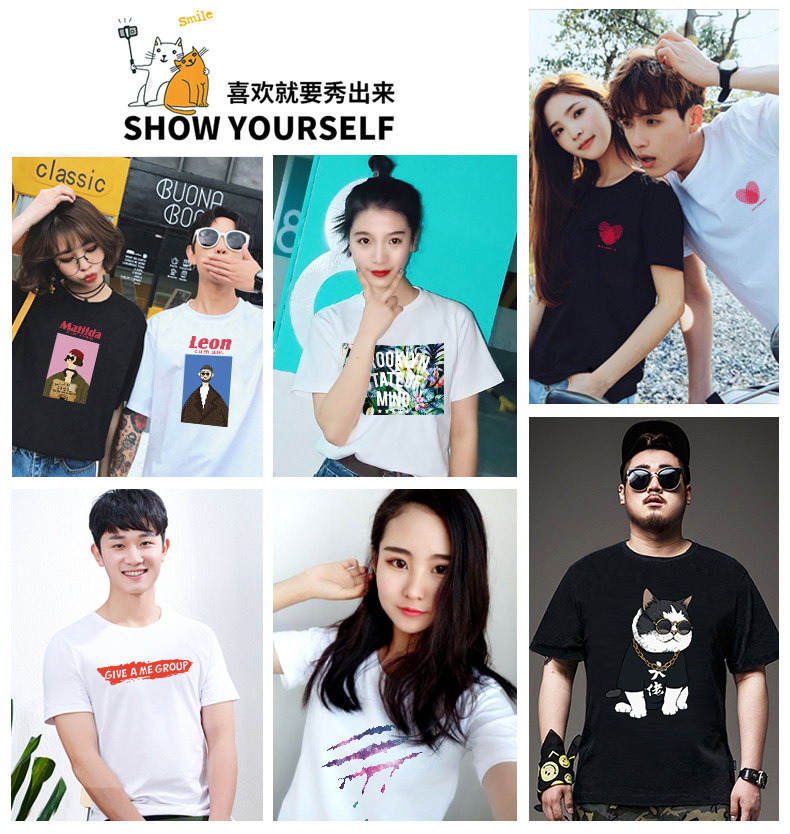•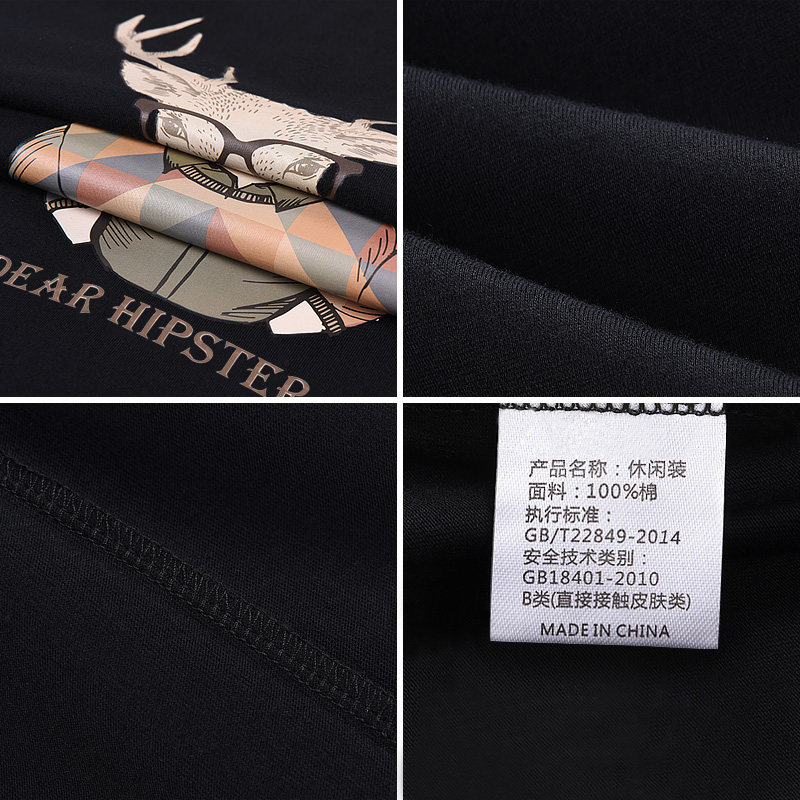### 短袖男2019新款潮牌t恤男士韩版潮流宽松ins嘻哈大码男装半袖衣服

252824 ชิ้น

฿ 323.61 69

• 品牌 : 南极人
• 材质 : 棉
• 棉含量 : 95%以上
• 尺码 : 3XL 165/S 170/M 175/L 180/XL 185/2XL 4XL 5XL 6XL
• 面料分类 : 棉毛布
• 花型图案 : 渐变
• 领型 : 圆领
• 颜色 : 图案颜色可备注 白色(百鬼夜行黑)+黑色(大佬猫) 白色(天太热)+黑色(大佬猫) 白色(新星空)+黑色(星部落) 黑色(星部落)+黑色(NSN) 黑色(逍遥自在白中标+逍遥自在白背标)+黑色(熬夜修仙白中标+熬夜修仙白背标) 白色(新星空)+黑色(NSN) 白色(星部落)+白色(百鬼夜行黑) 白色(新星空)+白色(铁三角) 白色(新星空)+黑色(HOT) 灰色(流行音乐)+黑色(吃鸡猫) 黑色(若水胸标+上善若水背标)+白色(百鬼夜行黑) 白色(天太热)+白色(永不言弃) 白色(一帆风顺)+黑色(百鬼夜行白) 白色(天太热)+黑色(星部落) 白色(一帆风顺)+皮粉色(烟斗男) 白色(大佬猫)+黑色(哈士奇) 姜黄色(三只鹤中标+三只鹤背标)+黑色(星部落) 黑色(星部落)+灰色(盘腿男孩) 白色(NSN)+白色(星部落) 黑色(铁三角)+黑色(never) 白色(HOT)+黑色(星部落) 黑色(HOT)+白色(热带风) 白色(热带风)+姜黄色(瓶盖儿) 白色(三只鹤中标+三只鹤背标)+姜黄色(鹤舞) 黑色(蒸蒸日上胸标+蒸蒸日上背标)+皮粉色(丛林狗) 白色(三只鹤中标+三只鹤背标)+灰色(天太热) 白色(HOT)+白色(椰子树) 图案颜色随机发(3件装纯棉短袖) 姜黄色(若水胸标+上善若水背标)+黑色(莲花) 白色(HOT)+白色(夏日风情) 黑色(蒸蒸日上胸标+蒸蒸日上背标)+白色(HOT) 白色(HOT)+皮粉色(元气猫) 黑色(逍遥自在白中标+逍遥自在白背标)+黑色(六尘不改白) 黑色(熬夜修仙白中标+熬夜修仙白背标)+黑色(瓶盖儿) 黑色(方框可乐男)+黑色(守望小熊白) 黑色(时差)+黑色(爱情契约胸标) 白色(naughty)+黑色(supoeme) 黑色(空白)+白色(空白) 黑色(拳击猫)+黑色(BURN) 白色(大佬猫)+黑色(吃鸡猫) 黑色(方框可乐男)+黑色(perfect) 黑色(悟禅白)+黑色(碳画蝙蝠) 白色(丛林狗)+红色(大佬猫) 白色(三人行)+黑色(新机器猫) 白色(music)+黑色(雕像) 黑色(方框可乐男)+黑色(雕像) 黑色(时尚之都)+黑色(A+W白) 黑色(时尚之都)+黑色(莲花) 黑色(layer)+黑色(seeyou) 黑色(佛系白)+黑色(小佛) 白色(BIUBIU)+黑色(A+W白) 黑色(五星阵中标+五星阵背标)+藏青(六尘不改白) 白色(HOT)+藏青(守望小熊白) 灰色(百鬼夜行黑)+藏青(笑笑)
• 袖型 : 常规
• 货号 : NJRDXA15
• 细分风格 : 潮
• 基础风格 : 青春流行
• 品牌类型 : 国内知名品牌
• 适用季节 : 夏季
• 上市年份季节 : 2018年夏季
• 袖长 : 短袖
• 厚薄 : 常规
• 适用场景 : 日常
• 版型 : 修身
• 款式细节 : 印花
• 服饰工艺 : 免烫处理
• 适用对象 : 青少年
• 印花主题 : 色彩世界
• 销售渠道类型 : 纯电商(只在线上销售)
• 材质成分 : 棉100%